# Adjoints and Inconsistency: A Questionable Test

#### (A new question of the week)

Last week we looked at how the adjugate matrix can be used to find an inverse. (This was formerly called the [classical] adjoint, a term that is avoided because it conflicts with another use of the word, but is still used in many sources.) I posted that as background for the question we’ll look at here.

## Testing consistency with the adjugate matrix

This question came from Rohit, in early March:

In our textbook, it is mentioned that while solving system of linear equation by matrix method, if |A| = 0, we then calculate (adj A)B. But why? How calculating (adj A)B tells us solution of system of linear equation??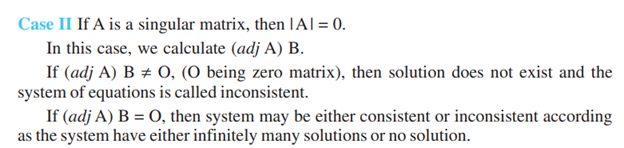This image appears to come from an NCERT textbook from India (chapter 4.7.1, page 32 of the pdf). The topic is solving a system of equations: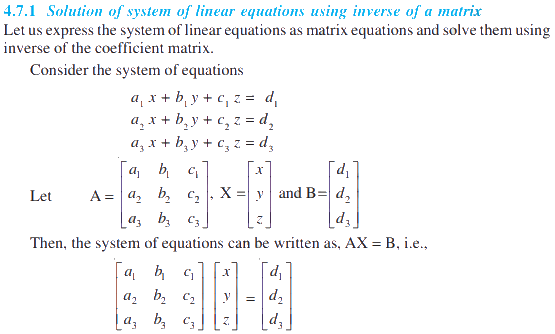We saw last week how the inverse of a matrix can be used to solve a system of equations, and that the inverse of an invertible matrix can be found (somewhat inefficiently) as $$\frac{1}{\det(\mathbf{A})}adj(\mathbf{A})$$, where $$adj(\mathbf{A})$$ is the adjugate (or “adjoint”) matrix, the transpose of the matrix of cofactors. This is how the inverse is found in this book.

(Note that the book specifies A as a 3×3 matrix, and X and B as 3×1 column vectors (matrices), representing three equations in three unknowns. The general idea of using the inverse matrix applies more broadly, but in this context, using the adjugate to find the inverse is not entirely unreasonable. For larger matrices, as we saw, the complexity of the calculation becomes prohibitive.)

Here is Case I, in which the system has a unique solution: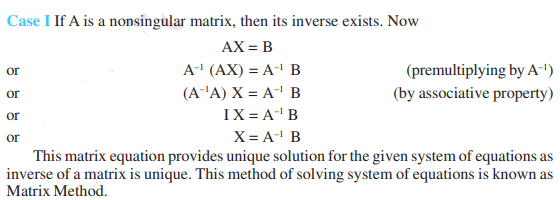But in Case II, when the determinant is 0, we can’t divide by it to find the inverse; instead, the book tells us in effect to skip the division, and just multiply by the adjugate itself. How does this tell us anything?

### Is it true? Is it provable?

Hi Rohit,

If A is a square matrix, adj(A) is the transpose of the cofactor matrix of A.  If A is singular, Ax = b must either be inconsistent, or consistent but having infinitely many solutions, so the second statement, that if (adj A)B = 0, then A must be either inconsistent or have infinitely many statements, just repeats the previous sentence and there is nothing to prove.

That is, the last case listed just says we’ve learned nothing beyond what we know merely because the determinant is zero. This test doesn’t apply, and we need some other way to determine whether there are any solutions. (But we aren’t told about any alternative test.)

The only content is the first statement, that if (adj A)B ≠ 0, then A must be inconsistent.  It’s not hard to show this with examples, but proving it in general is more difficult that I originally thought.  About the only information available on (adj A) is that for an invertible matrix A,

where I is the identity matrix, so that

In fact, it is true that $$(adj \mathbf{A})\mathbf{A}=\det(\mathbf{A})\mathbf{I}$$ regardless of whether A is invertible; this was shown last time, though without emphasizing this case. In the product on the LHS, every element on the main diagonal is the sum of elements of the corresponding column of A with their cofactors, and is therefore equal to the determinant; every element off the diagonal is 0.

I suspect his initial difficulty in proving the claim comes from looking at the adjugate from a modern perspective, focusing on row reduction methods; we’ll be proving it later.

If A is singular, and B is in the range (or column space) of A, then examples show that (adj A)B = 0, but if B is not in the column space, then examples show that (adj A)B ≠ 0, but I don’t see how to prove this.

The column space is the set of column matrices B that can be obtained by multiplying A by any column matrix X. So if B is in the column space, the system has at least one solution.

It is very easy to get one’s mind turned around here. The claim to be proved is not that if $$\mathbf{A}\mathbf{X}=\mathbf{B}$$ has a solution, then $$adj(\mathbf{A})\mathbf{B}\ne\mathbf{0}$$, but that if $$adj(\mathbf{A})\mathbf{B}\ne\mathbf{0}$$, then $$\mathbf{A}\mathbf{X}=\mathbf{B}$$ has a solution. We’ll see such a mistake in a textbook, below! And it took me forever to get my head around all this!

When A is non-singular, then if we write the n×2n augmented matrix [A : I] and reduce the left half to reduced row echelon form, then the right half becomes A-1, which is (1/|A|) (adj A),  but if A is singular and the left side is put in reduced row echelon form, the right side is not a scalar multiple of (adj A).

This is how we find an inverse by row reduction (as we also saw last week); here we see what the failure to find an inverse looks like in terms of the adjugate:

For example, if

    [ 1 2 3 ]                  [ 3  3 -3 ]
A = [ 4 5 6 ] , then   adj A = [-6 -6  6 ]
[ 5 7 9 ]                  [ 3  3 -3 ]

(row 3 is the sum of the first two rows, so A is singular), then row-reducing

[ 1 2 3 : 1 0 0 ]           [ 1  0 -1 : 0  7/3 -5/3 ]
[ 4 5 6 : 0 1 0 ]   gives   [ 0  1  2 : 0 -5/3  4/3 ]
[ 5 7 9 : 0 0 1 ]           [ 0  0  0 : 1   1   -1  ] ,

so the right half is not a scalar multiple of adj A.

We didn’t obtain I on the left, so what we have on the right is not an inverse (there is none). Now, what if we make a consistent system with this matrix A?

However, [ 1 1 1 ]T is a solution of

[ 1 2 3 ][ x ]   [  6 ]
[ 4 5 6 ][ y ] = [ 15 ]
[ 5 7 9 ][ z ]   [ 21 ],

and we find that

        [  6 ]   [ 0 ]                  [  6 ]   [  3 ]
(adj A) [ 15 ] = [ 0 ]    while (adj A) [ 15 ] = [ -6 ]
[ 21 ]   [ 0 ]                  [ 20 ]   [  3 ] ,

so this example bears out the claim of the second statement.

In the first example, with $$\mathbf{B}=\begin{bmatrix}6\\15\\21\end{bmatrix}$$, $$adj(\mathbf{A})\mathbf{B}=\mathbf{0}$$, and there are infinitely many solutions, one of which is $$\begin{bmatrix}1\\1\\1\end{bmatrix}$$; this is compatible with the second statement, which says we can’t be sure.

In the second example, with $$\mathbf{B}=\begin{bmatrix}6\\15\\20\end{bmatrix}$$, $$adj(\mathbf{A})\mathbf{B}\ne\mathbf{0}$$, and there is no solution, as implied by the first statement.

But is this always so? We don’t know yet. In practice it doesn’t really matter:

However, I don’t see why anyone would want to use this criterion to determine that AX = B is inconsistent.  Computing the adjugate matrix is an enormous amount of work: if A is n×n, adj A requires computing n2 (n-1)×(n-1) determinants.  That is far more work than simply using Gaussian elimination.

If we used the adjugate to find the inverse (which is not as hard in the 3×3 case as for larger matrices), then we have it sitting around to use in this test. But using Gaussian elimination to solve the system is little more work than using it just to find the inverse, and it gives us a simpler criterion for inconsistency.

We’ll set aside for now the question of whether the test as stated is true, because Rohit’s question turns out to be focused on a particular point.

## Comparing sources

After a technical problem disrupted the thread, Rohit restated the question, making his issue clearer:

I did not understand third point. In some book, it is written that if |A| = 0 and (adj A)B = 0 the system gives us infinite many solution i.e. it is consistent, but in our textbook it is written that it may be consistent or inconsistent. Please clarify this.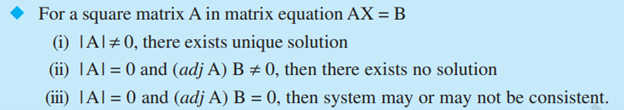This gives us a little more context. The new image appears to come from a similar textbook, namely the last page of this pdf. This version does not explicitly mention, in case (iii), that “consistent” means “infinitely many solutions” (which I describe as “too consistent”); but otherwise it is equivalent to the first.

Rohit also restated the question a third time in another thread, due to the technical problems, quoting yet another source, apparently quoting from StackExchange, with a different numbering of the same cases:

For a system of equations in matrix form AX = B,

If |A| ≠ 0, there exists a unique solution X = A−1B

That is fine, I understand this.

If |A| = 0,

Case 1: (adj A)⋅B ≠ O

then solution does not exist and the system of equations is called inconsistent.

Case 2: (adj A)⋅B = O

then system may be either consistent or inconsistent according as the system have either infinitely many solutions or no solution.

My doubt is: If |A| = 0 and (adj A)b ≠ 0 then the system is inconsistent clearly. If |A| = 0 and (adj A)b = 0 how can I reach the conclusion that “system may or may not be consistent according as the system have either infinitely many solutions or no solution” from |A|x=(adj A)b.

This narrows our focus to the last case. He sees this as a contradiction between books. As I read them, all three say the same thing; we haven’t yet seen “some book” that says that if $$(adj\mathbf{A})\mathbf{B}=\mathbf{0}$$, there must be infinitely many solutions. (We will.)

Doctor Rick responded:

I see that you want to focus on case 2. Doctor Fenton did answer this: He said,

If A is a square matrix, adj(A) is the transpose of the cofactor matrix of A.  If A is singular, Ax = b must either be inconsistent, or consistent but having infinitely many solutions, so the second statement, that if (adj A)B = 0, then A must be either inconsistent or have infinitely many solutions, just repeats the previous sentence and there is nothing to prove.

In other words, the statement in Case 2 merely repeats what we already know, that the system must be either consistent or inconsistent; it adds nothing new that we would need to prove. Let me put that another way: The claim is that

If |A| ≠ 0 then the system AX = B has a unique solution.

If |A| = 0 and (adj A)B ≠ 0 then the system is inconsistent and has no solutions.

If |A| = 0 and (adj A)B = 0 then there is insufficient information to tell whether the system is inconsistent or has infinitely many solutions. These cases must be distinguished by other means.

The real issue seems to be what he said in the second thread, which can be answered by examples:

You also showed that another source says that if |A| = 0 and (adj A)B = 0 the system has infinitely many solutions, thus disagreeing with what was said above. Is this what you are asking about also — which claim is correct?

If so, consider two very simple examples, in which A is reduced to diagonal form:

1. [1 0 0] [x]   
[0 1 0] [y] = 
[0 0 0] [z]   

2. [1 0 0] [x]   
[0 0 0] [y] = 
[0 0 0] [z]   

In each case, (a) Does the system AX = B have a unique solution, or an infinite number of solutions, or is it inconsistent (no solutions)? (b) What are |A| and (adj A)B?

These examples will demonstrate that, when $$(adj\mathbf{A})\mathbf{B}=\mathbf{0}$$, both outcomes are possible.

## Examining an erroneous textbook

Rohit carried out the work for each case:

Sir, what you said in the last, I solved it: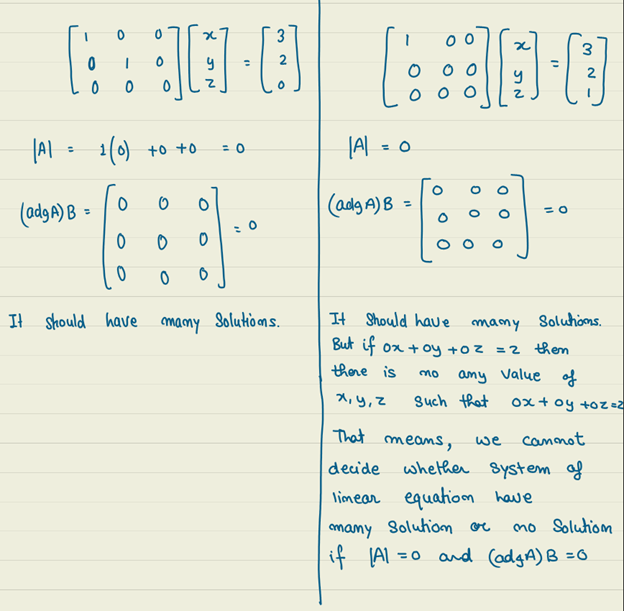So the two examples show that when $$|\mathbf{A}|=0$$ and $$(adj\mathbf{A})\mathbf{B}=\mathbf{0}$$, it is possible that either there are no solutions, or infinitely many. The first equation represents the system $$\left\{\begin{matrix}x=3\\y=2\\0=0\end{matrix}\right.$$ which has infinitely many solutions, $$\{3,2,z\}$$ for any z. The second equation represents the system $$\left\{\begin{matrix}x=3\\0=2\\0=1\end{matrix}\right.$$ which has no solutions.

But Rohit continued,

I think I get it. But I had read in Rd Sharma’s book that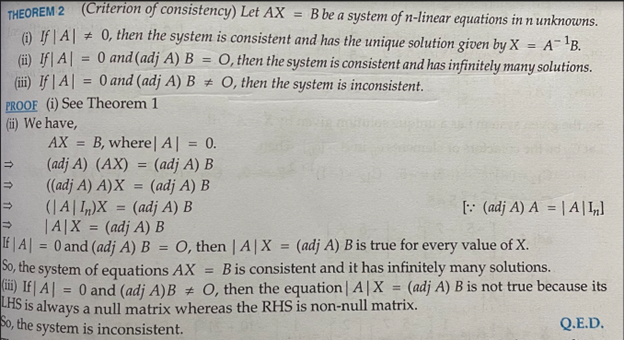and author proves it also. Is above proof wrong? If yes, then how we can prove it theoretically?

Now we finally see the book in which an incorrect statement is made (called case (ii) this time). And there is a “proof”, so we can have more to talk about!

### Find the fallacy

Doctor Rick replied:

I see that I was right, and you are indeed writing because two sources give contradictory information. Thanks for showing us the other source, including its proof; that is a great help. Here is the part of the proof that we’re talking about (part ii):

Theorem 2 (Criterion of consistency) Let AX = B be a system of n linear equations in n unknowns.

(ii) If |A| = 0 and (adj A)B = 0, then the system is consistent and has infinitely many solutions.

PROOF

(ii) We have,

AX = B, where |A| = 0.

If |A| = 0 and (adj A)B = O, then |A|X = (adj A)B is true for every value of X.

So, the system of equations AX = B is consistent and it has infinitely many solutions.

Can you find the flaw? It’s well hidden, but obvious once you see it.

Here is the reasoning I see in the proof:

If AX = B, then |A|X = (adj A)B.

If |A| = 0 and (adj A)B = O, then the conclusion above, |A|X = (adj A)B, is true.

Therefore the premise must be true: AX = B, so the system has solutions.

Is this good reasoning? NO! It boils down to the claim that

P implies Q

Q is true

Therefore P is true

This is the logical fallacy of affirming the consequent.

This fallacy is mentioned in our post Patterns of Logical Argument. It is also called the Fallacy of the Converse.

Note too that since the proof assumed that AX = B, the most that could have been claimed is that the conclusion is true for every value of X such that AX = B — not for every value of X, as claimed. If the system is inconsistent, then there is no such X, and whatever has been proved is true vacuously. Thus the result is consistent with the system being inconsistent, if I may put it that way.

But the fundamental error is affirming the consequent, or confusing sufficient conditions with necessary conditions. This is a good lesson to learn, but finding an error in a textbook is not the way we want to learn it!

The fact that the pattern of reasoning is fallacious tells us that the proof is wrong; the examples we looked at show that the theorem as stated is wrong. (These are two different things; it is possible to have a bad proof of a true statement!)

Rohit was satisfied:

Thank you sir, now I understand fully. Thanks a lot 🙂

Now Doctor Fenton rejoined the discussion, looking for a proof of the theorem in its (apparently) correct form, namely that if $$(adj\mathbf{A})\mathbf{B}\ne\mathbf{0}$$, there can be no solutions.

I have only seen the adjugate matrix in the context of computing the inverse matrix, in which case both A and adj A are invertible, and the equation AX = B has a unique solution for every B in Rn, and adj A is |A|A-1.  I have not seen any discussion of the adjugate when A is a singular square matrix.

For any matrix, there is a nullspace N(A) and a column space R(A) (also called the range of the matrix as a linear transformation).  If A is singular, then N(A) is non-trivial, with some positive dimension k (called the nullity of A), and the column space has a positive dimension called the rank r of A.  The Rank-Nullity Theorem says that r + k = n (if A is an n×n matrix), so that the column space is a proper subspace of Rn.  Every vector (column matrix) B in the column space is the image AX of some vector X in Rn, B = AX.  That is, the equation AX = B is consistent if and only if B is in the column space of A.  Since the column space is not all of Rn, AX = B will be consistent for some B and inconsistent for others.  For any B in the column space, AX = B will have infinitely many solutions (if X is one solution of AX = NB, then so is X + Y, when Y is any vector in the nullspace N(A)).

The nullspace of matrix A is the set of solution vectors X of $$\mathbf{A}\mathbf{X}=\mathbf{0}$$; the column space of matrix A is the set of all values of $$\mathbf{A}\mathbf{X}$$. Since we are talking about a matrix whose determinant is 0, it is singular, so its nullspace is not just $$\{\mathbf{0}\}$$, and its column space does not include all vectors. So some equations $$\mathbf{A}\mathbf{X}=\mathbf{B}$$ are consistent with infinitely many solutions (when B is in the column space), and others are inconsistent (when B is not). It could only have a unique solution if A were singular.

All of this is known without considering the adjugate of A.  The only real claim being made here is that if B is a vector such that (adj A)B ≠ 0, then AX = B is inconsistent.  (We already knew that AX = B will either be inconsistent, or consistent with infinitely many solutions.)  So essentially, the claim is that if (adj A)B ≠ 0, then B must not be in the column space.

We are trying to prove that, given that $$\det(\mathbf{A})=0$$, if $$(adj\mathbf{A})\mathbf{B}\ne\mathbf{0}$$, then the equation $$\mathbf{A}\mathbf{X}=\mathbf{B}$$ is necessarily inconsistent; that is, there are no solutions. This statement is equivalent to its contrapositive: If $$\mathbf{A}\mathbf{X}=\mathbf{B}$$ has a solution, then $$(adj \mathbf{A})\mathbf{B}=\mathbf{0}$$.

But we know that $$(adj\mathbf{A})\mathbf{A}=\det(\mathbf{A})\mathbf{I}$$. Therefore since $$\det(\mathbf{A})=0$$, $$(adj\mathbf{A})\mathbf{A}=\mathbf{0}$$. Now, if there is a solution to $$\mathbf{A}\mathbf{X}=\mathbf{B}$$, it follows that $$(adj \mathbf{A})(\mathbf{A}\mathbf{X})=((adj \mathbf{A})\mathbf{A})\mathbf{X}=\mathbf{0}\mathbf{X}=\mathbf{0}$$ Therefore, we must have $$(adj \mathbf{A})\mathbf{B}=\mathbf{0}$$.

This proves the claim.

To put it another way, if $$(adj \mathbf{A})\mathbf{B}\ne\mathbf{0}$$, then B is not in the column space of A.

But as I pointed out earlier, this question can be completely determined by using elimination to write the augmented matrix [A:B] and reducing the left side to ref (row echelon form) or rref (reduced row echelon form).  If A is singular, then there will be at least one entire row of 0’s in the left nxn portion of the augmented matrix.  If the last column has a non-0 entry in the row with all 0’s in the coefficient part of the augmented matrix, then the system is inconsistent for that B.

We saw this solution method last time. Let’s demonstrate it using a couple equations using the matrix $$A=\begin{bmatrix}1&2&3\\4&5&6\\5&7&9\end{bmatrix}$$ that we saw above, whose adjugate is $$\begin{bmatrix}3&3&-3\\-6&-6&6\\3&3&-3\end{bmatrix}$$:

• For the equation $$\mathbf{A}\mathbf{X}=\begin{bmatrix}6\\15\\20\end{bmatrix}$$, we can row-reduce the augmented matrix like this: $$\begin{bmatrix}1&2&3&|&6\\4&5&6&|&15\\5&7&9&|&20\end{bmatrix}\rightarrow\begin{bmatrix}1&2&3&|&6\\0&1&2&|&3\\0&0&0&|&-1\end{bmatrix}$$ so the system is inconsistent.

In this case, using the adjugate calculated previously, we find that $$(adj \mathbf{A})\mathbf{B}=\begin{bmatrix}3&3&-3\\-6&-6&6\\3&3&-3\end{bmatrix}\begin{bmatrix}6\\15\\20\end{bmatrix}=\begin{bmatrix}3\\-6\\3\end{bmatrix}\ne\mathbf{0}$$ so that, according to the theorem, the system must be, in fact, inconsistent.

• For the equation $$\mathbf{A}\mathbf{X}=\begin{bmatrix}6\\15\\21\end{bmatrix}$$, we can row-reduce the augmented matrix like this: $$\begin{bmatrix}1&2&3&|&6\\4&5&6&|&15\\5&7&9&|&21\end{bmatrix}\rightarrow\begin{bmatrix}1&2&3&|&6\\0&1&2&|&3\\0&0&0&|&0\end{bmatrix}$$ so the system has infinitely many solutions.

In this case, using the adjugate calculated previously, we find that $$(adj \mathbf{A})\mathbf{B}=\begin{bmatrix}3&3&-3\\-6&-6&6\\3&3&-3\end{bmatrix}\begin{bmatrix}6\\15\\21\end{bmatrix}=\begin{bmatrix}0\\0\\0\end{bmatrix}=\mathbf{0}$$ so that the theorem does not tell us whether the system should be inconsistent; we had to do the reduction to find out.

We have already seen an example where $$(adj \mathbf{A})\mathbf{B}=\mathbf{0}$$ but the system is inconsistent; here is a slightly less trivial-looking one:

For the equation $$\begin{bmatrix}1&2&3\\1&2&3\\1&2&3\end{bmatrix}\mathbf{X}=\begin{bmatrix}1\\2\\3\end{bmatrix},$$ we can row-reduce the augmented matrix like this: $$\begin{bmatrix}1&2&3&|&1\\1&2&3&|&2\\1&2&3&|&3\end{bmatrix}\rightarrow\begin{bmatrix}1&2&3&|&1\\0&0&0&|&1\\0&0&0&|&1\end{bmatrix}$$ so the system is inconsistent.

But the adjugate is $$\begin{bmatrix}0&0&0\\0&0&0\\0&0&0\end{bmatrix}$$, and we find that $$(adj \mathbf{A})\mathbf{B}=\begin{bmatrix}0&0&0\\0&0&0\\0&0&0\end{bmatrix}\begin{bmatrix}1\\2\\3\end{bmatrix}=\begin{bmatrix}0\\0\\0\end{bmatrix}=\mathbf{0}$$ which, again, is not enough to tell us anything.

### 1 thought on “Adjoints and Inconsistency: A Questionable Test”

1.Thankyou so much sir.

This site uses Akismet to reduce spam. Learn how your comment data is processed.